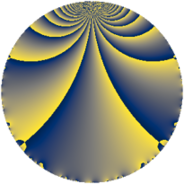Properties

 Label 8280.2.a.bdLevel $8280$ Weight $2$ Character orbit 8280.a Self dual yes Analytic conductor $66.116$ Analytic rank $1$ Dimension $2$ CM no Inner twists $1$

Related objects

Newspace parameters

 Level: $$N$$ $$=$$ $$8280 = 2^{3} \cdot 3^{2} \cdot 5 \cdot 23$$ Weight: $$k$$ $$=$$ $$2$$ Character orbit: $$[\chi]$$ $$=$$ 8280.a (trivial)

Newform invariants

 Self dual: yes Analytic conductor: $$66.1161328736$$ Analytic rank: $$1$$ Dimension: $$2$$ Coefficient field: $$\Q(\sqrt{17})$$ Defining polynomial: $$x^{2} - x - 4$$ Coefficient ring: $$\Z[a_1, \ldots, a_{7}]$$ Coefficient ring index: $$1$$ Twist minimal: no (minimal twist has level 2760) Fricke sign: $$1$$ Sato-Tate group: $\mathrm{SU}(2)$

$q$-expansion

Coefficients of the $$q$$-expansion are expressed in terms of $$\beta = \frac{1}{2}(1 + \sqrt{17})$$. We also show the integral $$q$$-expansion of the trace form.

 $$f(q)$$ $$=$$ $$q + q^{5} + ( -1 - \beta ) q^{7} +O(q^{10})$$ $$q + q^{5} + ( -1 - \beta ) q^{7} -2 q^{11} -2 q^{13} + ( 1 - \beta ) q^{17} + ( 2 + 2 \beta ) q^{19} + q^{23} + q^{25} + ( -3 + \beta ) q^{29} + ( 1 + 3 \beta ) q^{31} + ( -1 - \beta ) q^{35} + ( -1 - 3 \beta ) q^{37} + ( -1 + 3 \beta ) q^{41} + ( 4 - 4 \beta ) q^{47} + ( -2 + 3 \beta ) q^{49} + ( 5 + \beta ) q^{53} -2 q^{55} + ( -1 + \beta ) q^{59} + ( -8 + 4 \beta ) q^{61} -2 q^{65} + ( 7 - 3 \beta ) q^{67} + ( -3 + 7 \beta ) q^{71} -10 q^{73} + ( 2 + 2 \beta ) q^{77} + ( 4 - 6 \beta ) q^{79} + ( 1 - 3 \beta ) q^{83} + ( 1 - \beta ) q^{85} -4 \beta q^{89} + ( 2 + 2 \beta ) q^{91} + ( 2 + 2 \beta ) q^{95} -14 q^{97} +O(q^{100})$$ $$\operatorname{Tr}(f)(q)$$ $$=$$ $$2 q + 2 q^{5} - 3 q^{7} + O(q^{10})$$ $$2 q + 2 q^{5} - 3 q^{7} - 4 q^{11} - 4 q^{13} + q^{17} + 6 q^{19} + 2 q^{23} + 2 q^{25} - 5 q^{29} + 5 q^{31} - 3 q^{35} - 5 q^{37} + q^{41} + 4 q^{47} - q^{49} + 11 q^{53} - 4 q^{55} - q^{59} - 12 q^{61} - 4 q^{65} + 11 q^{67} + q^{71} - 20 q^{73} + 6 q^{77} + 2 q^{79} - q^{83} + q^{85} - 4 q^{89} + 6 q^{91} + 6 q^{95} - 28 q^{97} + O(q^{100})$$

Embeddings

For each embedding $$\iota_m$$ of the coefficient field, the values $$\iota_m(a_n)$$ are shown below.

For more information on an embedded modular form you can click on its label.

Label $$\iota_m(\nu)$$ $$a_{2}$$ $$a_{3}$$ $$a_{4}$$ $$a_{5}$$ $$a_{6}$$ $$a_{7}$$ $$a_{8}$$ $$a_{9}$$ $$a_{10}$$
1.1
 2.56155 −1.56155
0 0 0 1.00000 0 −3.56155 0 0 0
1.2 0 0 0 1.00000 0 0.561553 0 0 0
 $$n$$: e.g. 2-40 or 990-1000 Significant digits: Format: Complex embeddings Normalized embeddings Satake parameters Satake angles

Atkin-Lehner signs

$$p$$ Sign
$$2$$ $$-1$$
$$3$$ $$-1$$
$$5$$ $$-1$$
$$23$$ $$-1$$

Inner twists

This newform does not admit any (nontrivial) inner twists.

Twists

By twisting character orbit
Char Parity Ord Mult Type Twist Min Dim
1.a even 1 1 trivial 8280.2.a.bd 2
3.b odd 2 1 2760.2.a.m 2
12.b even 2 1 5520.2.a.bn 2

By twisted newform orbit
Twist Min Dim Char Parity Ord Mult Type
2760.2.a.m 2 3.b odd 2 1
5520.2.a.bn 2 12.b even 2 1
8280.2.a.bd 2 1.a even 1 1 trivial

Hecke kernels

This newform subspace can be constructed as the intersection of the kernels of the following linear operators acting on $$S_{2}^{\mathrm{new}}(\Gamma_0(8280))$$:

 $$T_{7}^{2} + 3 T_{7} - 2$$ $$T_{11} + 2$$ $$T_{13} + 2$$ $$T_{17}^{2} - T_{17} - 4$$

Hecke characteristic polynomials

$p$ $F_p(T)$
$2$ $$T^{2}$$
$3$ $$T^{2}$$
$5$ $$( -1 + T )^{2}$$
$7$ $$-2 + 3 T + T^{2}$$
$11$ $$( 2 + T )^{2}$$
$13$ $$( 2 + T )^{2}$$
$17$ $$-4 - T + T^{2}$$
$19$ $$-8 - 6 T + T^{2}$$
$23$ $$( -1 + T )^{2}$$
$29$ $$2 + 5 T + T^{2}$$
$31$ $$-32 - 5 T + T^{2}$$
$37$ $$-32 + 5 T + T^{2}$$
$41$ $$-38 - T + T^{2}$$
$43$ $$T^{2}$$
$47$ $$-64 - 4 T + T^{2}$$
$53$ $$26 - 11 T + T^{2}$$
$59$ $$-4 + T + T^{2}$$
$61$ $$-32 + 12 T + T^{2}$$
$67$ $$-8 - 11 T + T^{2}$$
$71$ $$-208 - T + T^{2}$$
$73$ $$( 10 + T )^{2}$$
$79$ $$-152 - 2 T + T^{2}$$
$83$ $$-38 + T + T^{2}$$
$89$ $$-64 + 4 T + T^{2}$$
$97$ $$( 14 + T )^{2}$$